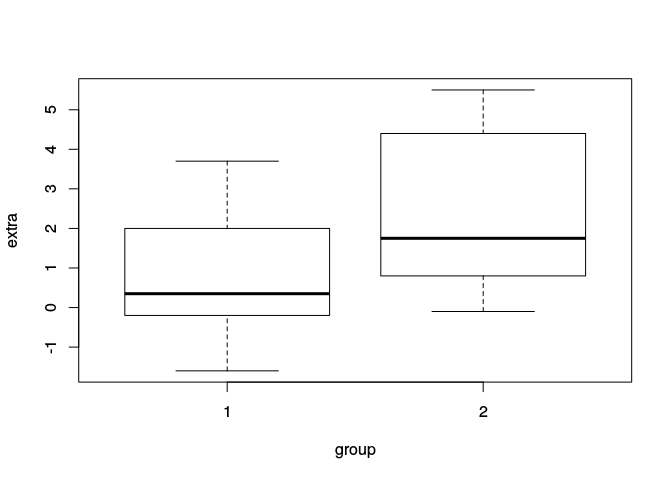# Contents

The material in this course requires R version 3.2 and Bioconductor version 3.2

stopifnot(
getRversion() >= '3.2' && getRversion() < '3.3',
BiocInstaller::biocVersion() == "3.2"
)

# 1 Example: t-test

t.test()

• x: vector of univariate measurements
• y: factor describing experimental design
• var.equal=TRUE: appropriate for relatively small experiments where no additional information available?
• formula: alternative representation, y ~ x.
head(sleep)
##   extra group ID
## 1   0.7     1  1
## 2  -1.6     1  2
## 3  -0.2     1  3
## 4  -1.2     1  4
## 5  -0.1     1  5
## 6   3.4     1  6
plot(extra ~ group, data = sleep)## Traditional interface
with(sleep, t.test(extra[group == 1], extra[group == 2]))
##
##  Welch Two Sample t-test
##
## data:  extra[group == 1] and extra[group == 2]
## t = -1.8608, df = 17.776, p-value = 0.07939
## alternative hypothesis: true difference in means is not equal to 0
## 95 percent confidence interval:
##  -3.3654832  0.2054832
## sample estimates:
## mean of x mean of y
##      0.75      2.33
## Formula interface
t.test(extra ~ group, sleep)
##
##  Welch Two Sample t-test
##
## data:  extra by group
## t = -1.8608, df = 17.776, p-value = 0.07939
## alternative hypothesis: true difference in means is not equal to 0
## 95 percent confidence interval:
##  -3.3654832  0.2054832
## sample estimates:
## mean in group 1 mean in group 2
##            0.75            2.33
## equal variance between groups
t.test(extra ~ group, sleep, var.equal=TRUE)
##
##  Two Sample t-test
##
## data:  extra by group
## t = -1.8608, df = 18, p-value = 0.07919
## alternative hypothesis: true difference in means is not equal to 0
## 95 percent confidence interval:
##  -3.363874  0.203874
## sample estimates:
## mean in group 1 mean in group 2
##            0.75            2.33

lm() and anova()

• lm(): fit linear model.
• anova(): statisitcal evaluation.
## linear model; compare to t.test(var.equal=TRUE)
fit <- lm(extra ~ group, sleep)
anova(fit)
## Analysis of Variance Table
##
## Response: extra
##           Df Sum Sq Mean Sq F value  Pr(>F)
## group      1 12.482 12.4820  3.4626 0.07919 .
## Residuals 18 64.886  3.6048
## ---
## Signif. codes:  0 '***' 0.001 '**' 0.01 '*' 0.05 '.' 0.1 ' ' 1
• Under the hood: formula: translated into model matrix, used in lm.fit().
• With (implicit) intercept 1, last coefficient of model matrix reflects group effect
• With intercept 0, contrast between effects of coefficient 1 and coefficient 2 reflect group effect
## underlying model, used in lm.fit()
model.matrix(extra ~ group, sleep)     # last column indicates group effect
##    (Intercept) group2
## 1            1      0
## 2            1      0
## 3            1      0
## 4            1      0
## 5            1      0
## 6            1      0
## 7            1      0
## 8            1      0
## 9            1      0
## 10           1      0
## 11           1      1
## 12           1      1
## 13           1      1
## 14           1      1
## 15           1      1
## 16           1      1
## 17           1      1
## 18           1      1
## 19           1      1
## 20           1      1
## attr(,"assign")
##  0 1
## attr(,"contrasts")
## attr(,"contrasts")$group ##  "contr.treatment" model.matrix(extra ~ 0 + group, sleep) # contrast between columns ## group1 group2 ## 1 1 0 ## 2 1 0 ## 3 1 0 ## 4 1 0 ## 5 1 0 ## 6 1 0 ## 7 1 0 ## 8 1 0 ## 9 1 0 ## 10 1 0 ## 11 0 1 ## 12 0 1 ## 13 0 1 ## 14 0 1 ## 15 0 1 ## 16 0 1 ## 17 0 1 ## 18 0 1 ## 19 0 1 ## 20 0 1 ## attr(,"assign") ##  1 1 ## attr(,"contrasts") ## attr(,"contrasts")$group
##  "contr.treatment"
• Covariate – fit base model containing only covariate, test improvement in fit when model includes factor of interest
fit0 <- lm(extra ~ ID, sleep)
fit1 <- lm(extra ~ ID + group, sleep)
anova(fit0, fit1)
## Analysis of Variance Table
##
## Model 1: extra ~ ID
## Model 2: extra ~ ID + group
##   Res.Df    RSS Df Sum of Sq      F   Pr(>F)
## 1     10 19.290
## 2      9  6.808  1    12.482 16.501 0.002833 **
## ---
## Signif. codes:  0 '***' 0.001 '**' 0.01 '*' 0.05 '.' 0.1 ' ' 1
t.test(extra ~ group, sleep, var.equal=TRUE, paired=TRUE)
##
##  Paired t-test
##
## data:  extra by group
## t = -4.0621, df = 9, p-value = 0.002833
## alternative hypothesis: true difference in means is not equal to 0
## 95 percent confidence interval:
##  -2.4598858 -0.7001142
## sample estimates:
## mean of the differences
##                   -1.58

genefilter::rowttests()

• t-tests for gene expression data
• useful for exploratory analysis, but statistically sub-optimal
• x: matrix of expression values
• features x samples (reverse of how a ‘statistician’ would represent the data – samples x features)

• fac: factor of one or two levels describing experimental design

Limitations

• Assumes features are independent
• Ignores common experimental design
• Ignores multiple testing

Consequences

• Poor estimate of between-group variance for each feature
• Elevated false discovery rate

# 2 Common experimental designs

• t-test: count ~ factor. Alternative: count ~ 0 + factor and contrasts
• covariates: count ~ covariate + factor
• Single factor, multiple levels (one-way ANOVA) – statistical contrasts: specify model as count ~ factor or count ~ 0 + factor
• Factorial designs – main effects, count ~ factor1 + factor2; main effects and interactions, count ~ factor1 * factor2. Contrasts to ask specific questions
• Paired designs: include ID as covariate (approximate, since ID is a random effect); limma approach: duplicateCorrelation()